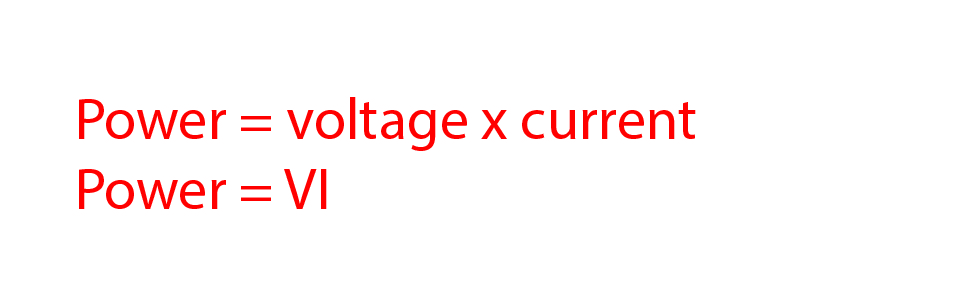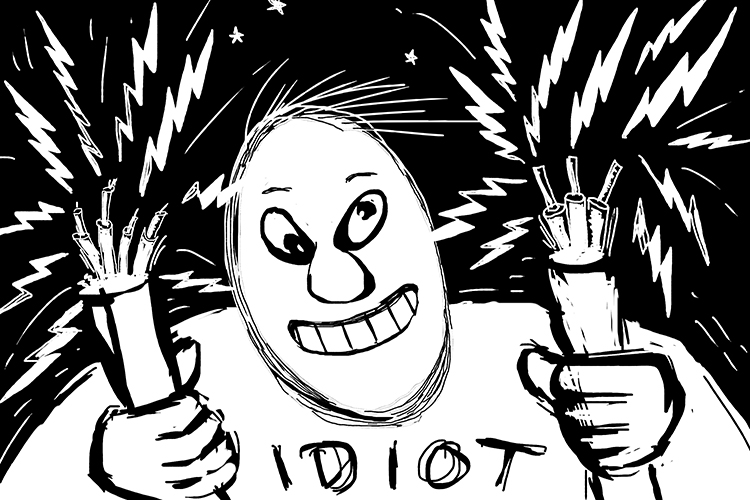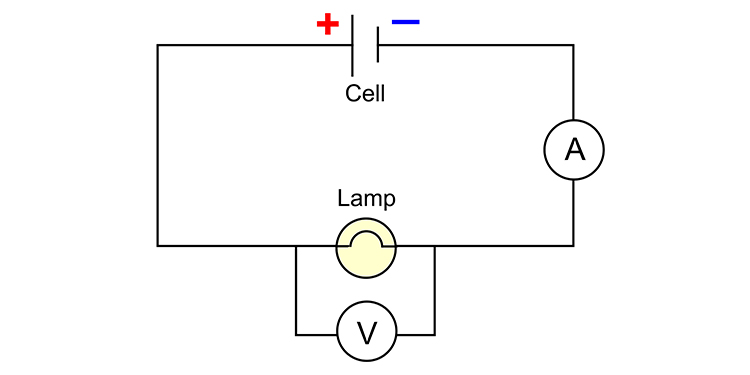# Electrical power

Electrical power is the rate or speed at which electrical energy is transferred in a circuit. It can also be thought of as the rate at which energy is consumed by an item in an electrical circuit. The formula for calculating electrical power is:Electrical power turned me into the village idiot.

NOTE:

Electrical power is measured in watts. One watt of electrical power is one joule per second.

Example 1

A lamp is rated at 12V, 6W. How many joules of energy are transferred by the lamp in 5 minutes? And what is the current passing through the lamp when it is connected to a 12V supply?

6 watts = 6 joules per second

5 minutes = 60 x 5 = 300 seconds

So the energy transferred = 300 x 6 = 1800 Joules

Power = voltage x current                            P = VI

Divide both sides by voltage to make current the subject,

\frac{Power}{voltage}=\frac{\strike{voltage}\times current}{\strike{voltage}}

So current I = P/V        6/12 = 0.5 Amps

Example 2

The circuit below shows where an ammeter and voltmeter are placed. If the ammeter reads 2A and the voltmeter reads 6V, what is the power of the lamp?